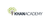Electrical engineering: Impedance

Product type
Total time

Electrical engineering: ImpedanceKhan Academy

Need more information? Get more details on the site of the provider.Description

The ratio of a sinusoidal voltage to a sinusoidal current is called "impedance". This is a generalization of Ohm's Law for resistors. We derive the impedance of a resistor, inductor, and capacitor. The inductor and capacitor impedance includes a term for frequency, so the impedance of these components depends on frequency.

Electrical engineering

Learn about electricity, circuit theory, and introductory electronics. We also have sections that let you get down and dirty with household items, from building your own robot to videos where we disassemble household items to see what's inside.

AC circuit analyisis

If we limit ourselves to input signals shaped like sines or cosines, we can develop a …

There are no frequently asked questions yet. If you have any more questions or need help, contact our customer service.

The ratio of a sinusoidal voltage to a sinusoidal current is called "impedance". This is a generalization of Ohm's Law for resistors. We derive the impedance of a resistor, inductor, and capacitor. The inductor and capacitor impedance includes a term for frequency, so the impedance of these components depends on frequency.

Electrical engineering

Learn about electricity, circuit theory, and introductory electronics. We also have sections that let you get down and dirty with household items, from building your own robot to videos where we disassemble household items to see what's inside.

AC circuit analyisis

If we limit ourselves to input signals shaped like sines or cosines, we can develop a whole AC analysis method to figure out what happens in circuits with changing signals. The methods are far simpler than solving a differential equation.

Topic: Science

Learn about all the sciences, from physics, chemistry and biology, to cosmology and astronomy, across hundreds of videos, articles and practice questions.

There are no reviews yet.